## 概述

1. 扩张树：用贪心，上至下递归分区方法

2. split function 选择最好的特征 $$j*$$ 和该特征最好的值 $$t*$$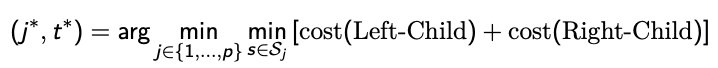Split s divide the current node into two children.

2D 的例子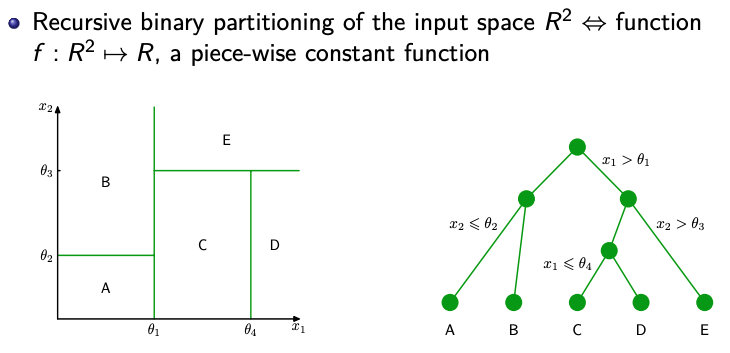## Splitting 规则

### 1. Regression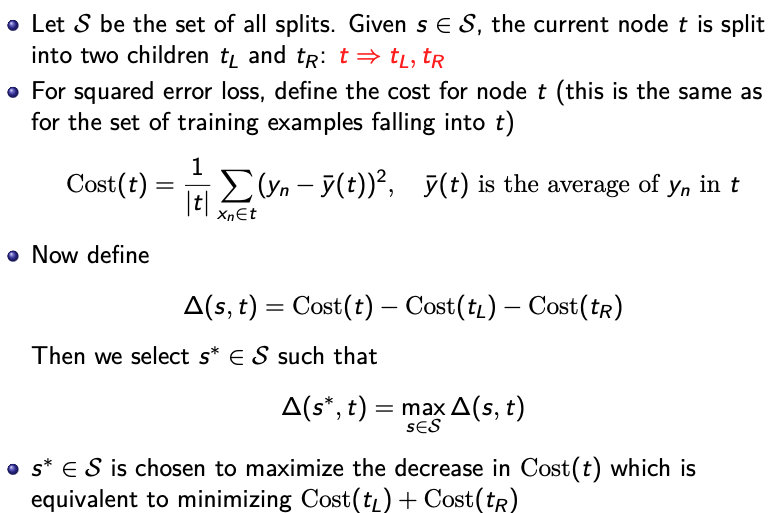### 2. Classification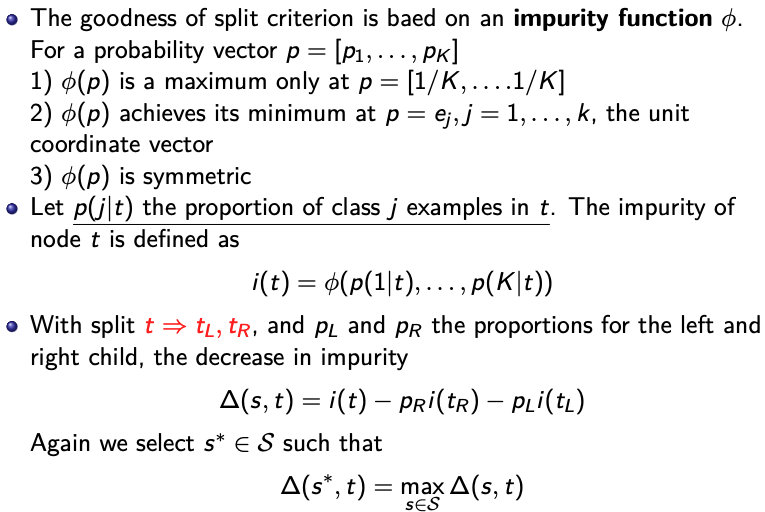1. 当所有样本都属于同一类时候 $$I$$ 取最小值. 即 $$I$$ 在点 $$(1,0,…,0),(0,1,…,0),…,(0,..,0,1)$$ 取最小值.

2. 当样本中每个类目下样本个数相同时I取最大值. 即 $$I$$ 在点 $$(1/k,..,1/k)$$ 取最大值.

## Prunning 剪枝

$$min \ \ \frac {1}{N} \sum^{\vert T \vert}_{m=1} \sum_{x_i \in {R_m}} L(y_i, w_m) + \alpha \vert T\vert$$

$$\vert T \vert$$ 是 termainal nodes 的总数

$$L(·, ·)$$ 是 loss function, 例如 $$L(yi, f (x_i)) = L(x_i,w_m) = (y_i − w_m)^2$$

$$w_m$$ 是与 $$R_m$$ 对应的预测值 $$\rightarrow$$ 也就是 $$R_m$$ 中训练集的平均值

## 算法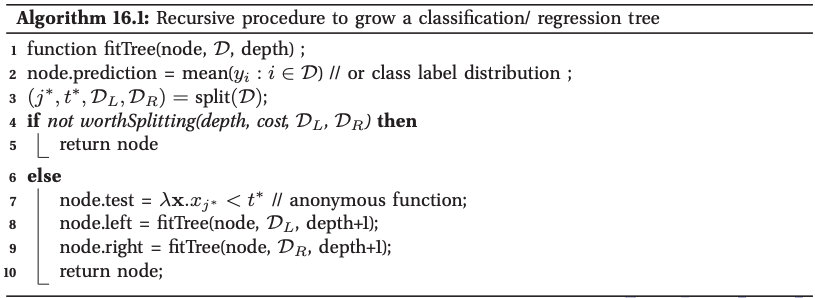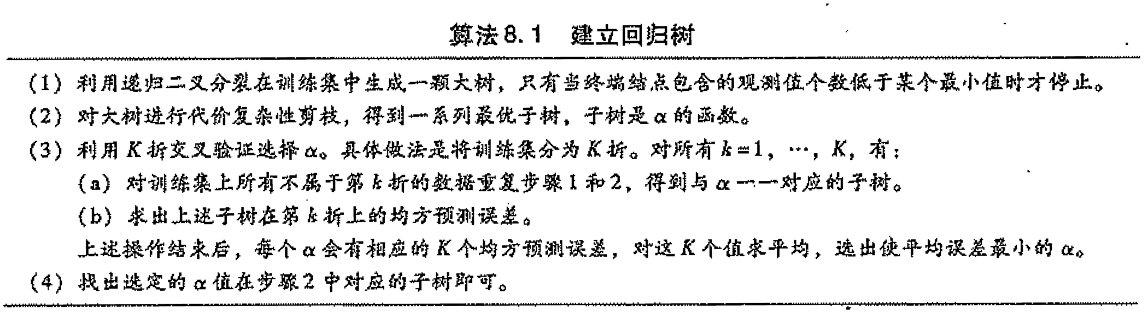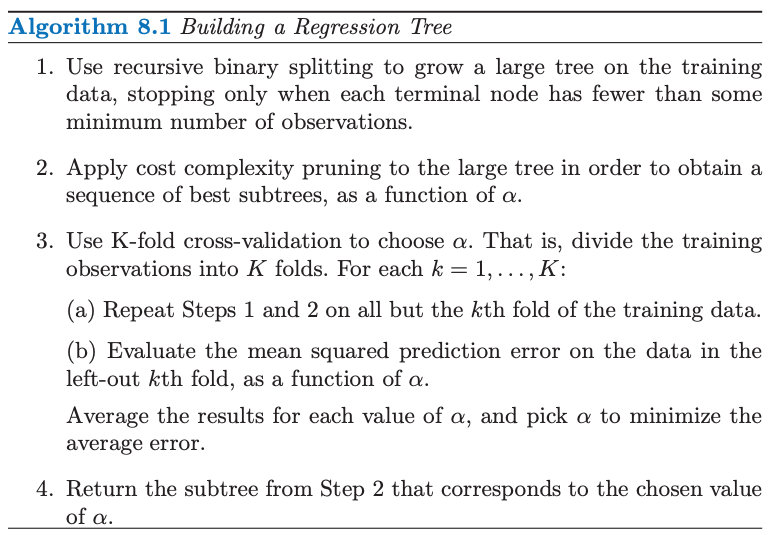Multiple trees:

• bagging 袋装法
• random forests 随机森林
• boosting 提升法

## boosting

• PAC learning framework (1990)
• AdaBoost methods (1996)
• gradient boosting (2000)

weak learner:
classifiers whose error rate is slightly better than random guessing

Boosting 改变训练样本的权重，产生一系列的分类器：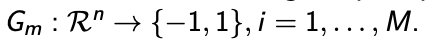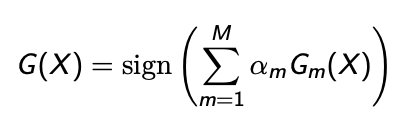$$\alpha_m$$：分类系数（由 boosting 算法计算得出）

### AdaBoost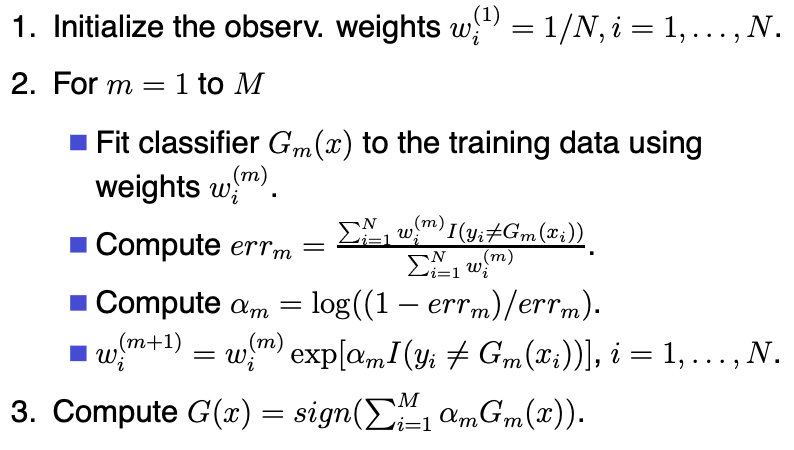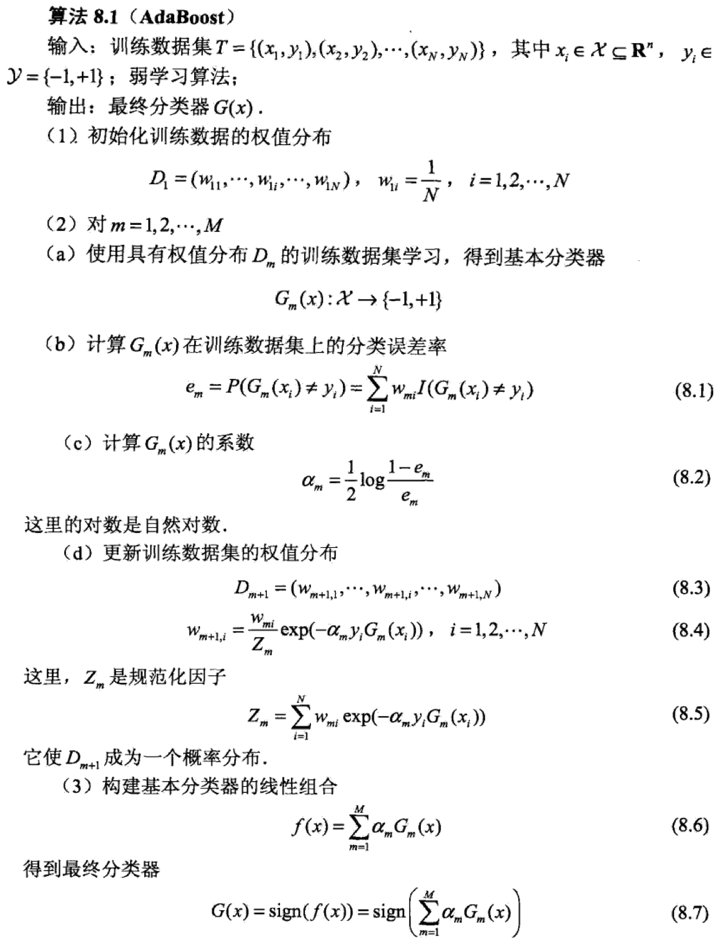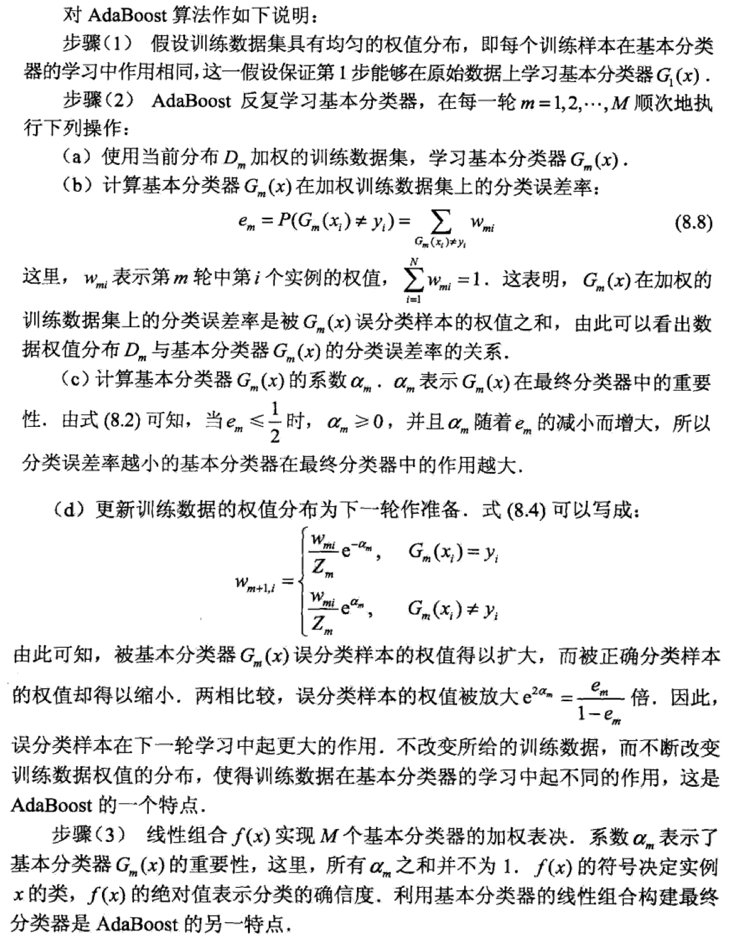## 随机森林

1. 随机采样数据点

2. 基于特征的子集分割节点

1. 样本的随机：从样本集中用Bootstrap随机选取n个样本

2. 特征的随机：从所有属性中随机选取K个属性，选择最佳分割属性作为节点建立CART决策树（泛化的理解，这里面也可以是其他类型的分类器，比如SVM、Logistics）

3. 重复以上两步m次，即建立了m棵CART决策树

4. 这m个CART形成随机森林，通过投票表决结果，决定数据属于哪一类（投票机制有一票否决制、少数服从多数、加权多数）

1. 如何选取K，可以考虑有N个属性，取K=根号N
2. 最大深度（不超过8层）
3. 棵数
4. 最小分裂样本树
5. 类别比例